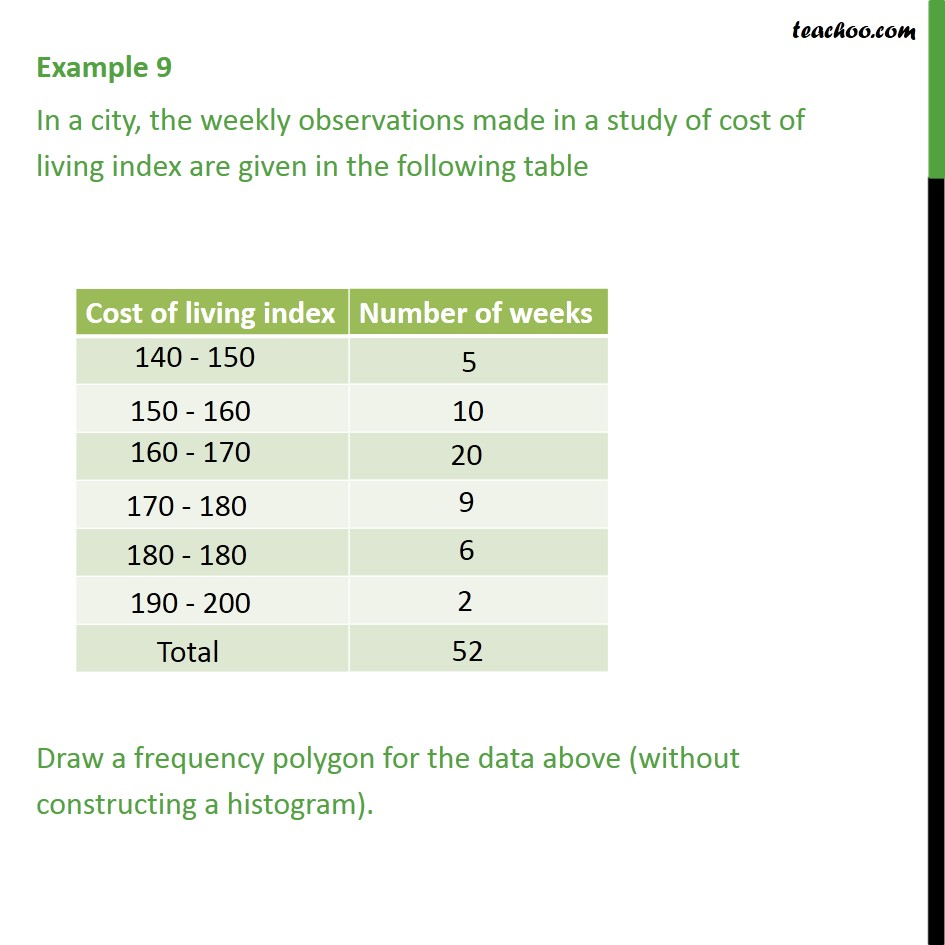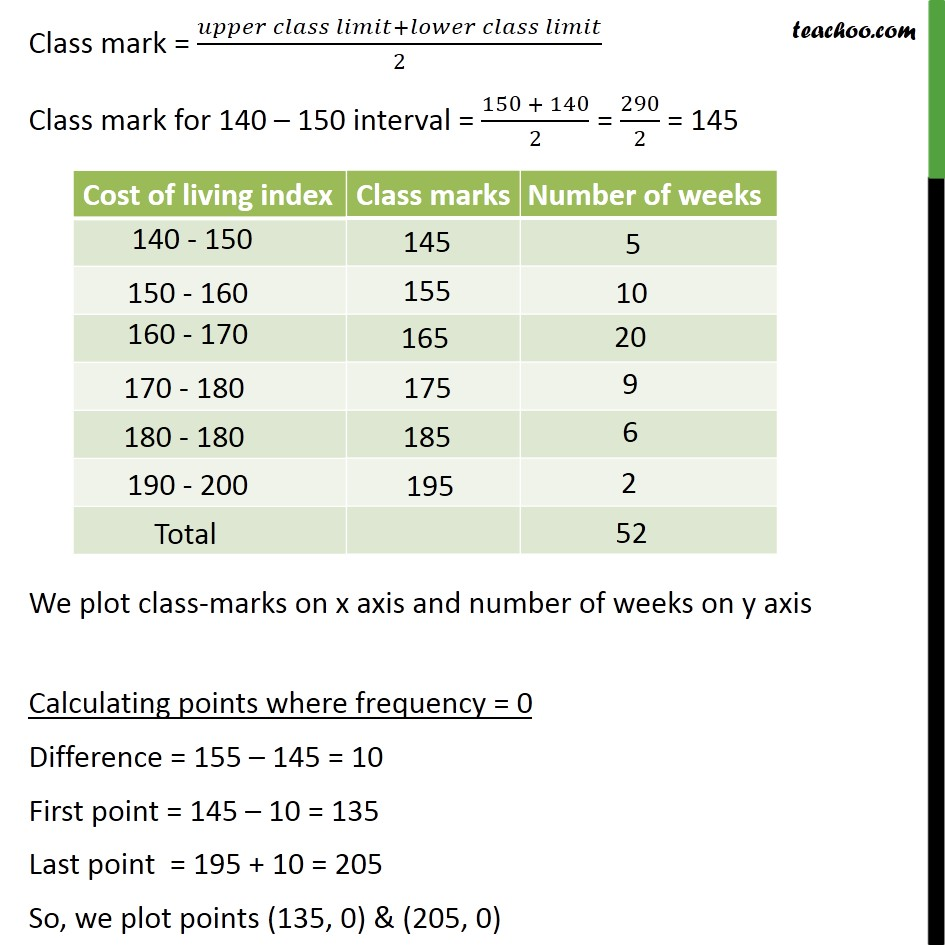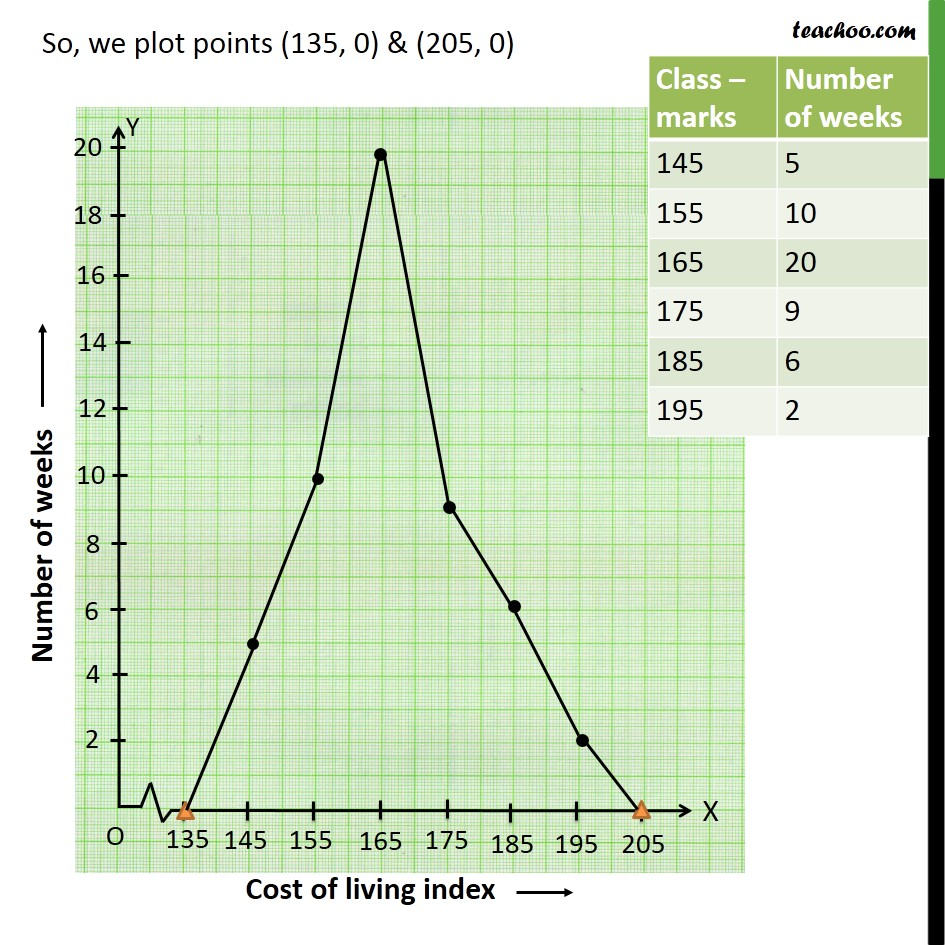Frequency polygon

Chapter 14 Class 9 Statistics
Concept wiseSolve all your doubts with Teachoo Black (new monthly pack available now!)

### Transcript

Example 9 In a city, the weekly observations made in a study of cost of living index are given in the following table Draw a frequency polygon for the data above (without constructing a histogram). Class mark = (𝑢𝑝𝑝𝑒𝑟 𝑐𝑙𝑎𝑠𝑠 𝑙𝑖𝑚𝑖𝑡+𝑙𝑜𝑤𝑒𝑟 𝑐𝑙𝑎𝑠𝑠 𝑙𝑖𝑚𝑖𝑡)/2 Class mark for 140 – 150 interval = (150 + 140)/2 = 290/2 = 145 We plot class-marks on x axis and number of weeks on y axis Calculating points where frequency = 0 Difference = 155 – 145 = 10 First point = 145 – 10 = 135 Last point = 195 + 10 = 205 So, we plot points (135, 0) & (205, 0)